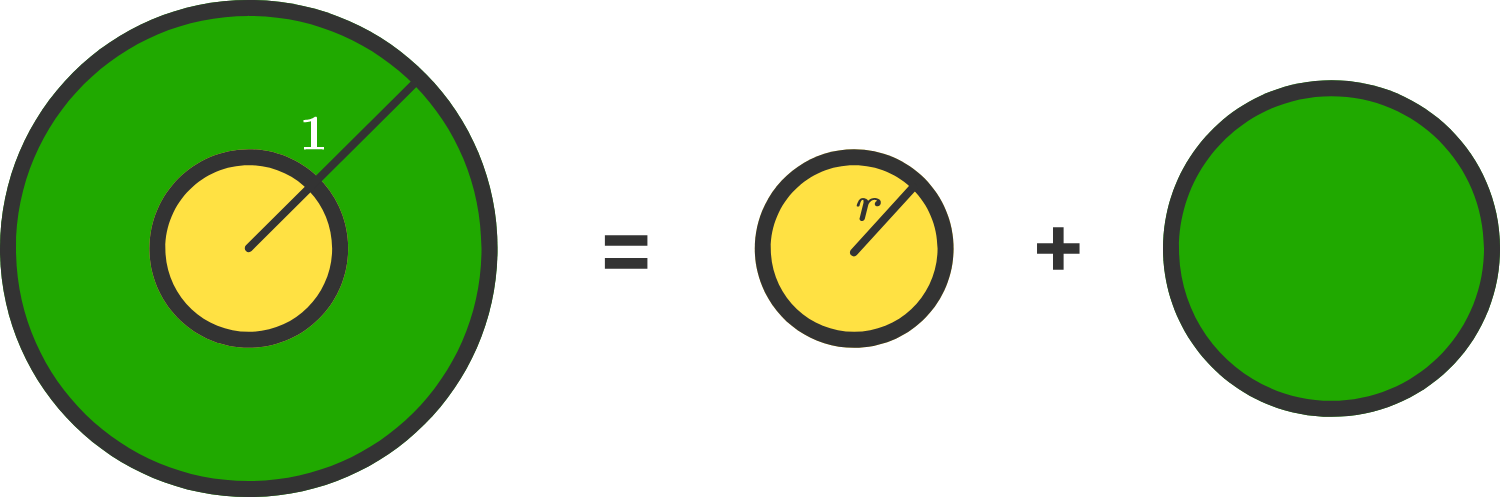# Reformed Disk

Calculus Level 3A unit disk (radius = 1) is partitioned into an inner circular region of radius $(r < 1)$ and an outer region consisting of the area between concentric circles of radii $r$ and 1 respectively.

The inner region is preserved, and the outer region is reformed into a circular disk with equivalent area. Thus, the combined area of the two resulting disks is equal to the area of the original unit disk.

Which value of $r$ (to 3 decimal places) maximizes the sum of the circumferences of the two final disks?

Note: Image might not be necessarily be up to scale.

×